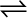7.33 The value of Kc for the reaction 3O2 (g)2O3 (g) is 2.0 ×10–50 at 25°C. If the equilibrium concentration of O2 in air at 25°C is 1.6 ×10–2, what is the concentration of O3?

The given reaction is:

It is given that

Then we have,

Hence, the concentration of